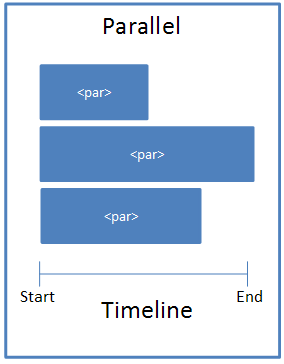This element describes the Parallel time node which can be activated along with other parallel time node containers. Conceptually it can be thought of as follows:[Example: Consider a simple animation with a blind entrance. The <par> element should be used as follows:

<p:tnLst>

<p:par>

<p:cTn id="1" dur="indefinite" restart="never" nodeType="tmRoot">

<p:childTnLst>

<p:seq concurrent="1" nextAc="seek">

...

</p:seq>

</p:childTnLst>

</p:cTn>

</p:par>

</p:tnLst>

</p:timing>

End Example]

 Parent Elements childTnLst (§4.6.25); subTnLst (§4.6.78); tnLst (§4.6.87)

 Child Elements Subclause cTn (Common Time Node Properties) §4.6.33

The following XML Schema fragment defines the contents of this element:

<complexType name="CT_TLTimeNodeParallel">

<sequence>

<element name="cTn" type="CT_TLCommonTimeNodeData" minOccurs="1" maxOccurs="1"/>

</sequence>

</complexType>